# Exploring metal subgenres with Python¶

An exploration. We invariably end up doing some processing that we don't directly need, but that would be useful for a future analysis, or one of your own. Choose your own adventure.

## Requirements¶

This notebook was written using Python 3.5. If you're following along with this notebook, you should have roughly up to date versions of the following libraries (installable via pip, conda, or your package manager of choice):

• requests
• BeautifulSoup4
• pandas
• numpy
• matplotlib
• seaborn (optional)
• scikit-learn
• wordcloud
• networkx
• circos

# Part I: Scraping, cleaning, & clustering¶

## Scraping¶

The data can be obtained by running the script MA_scraper.py. It scrapes M-A for a listing of band names alphabetically (as seen here for example). The site uses AJAX to retrieve band names and display them in the page. It does so in chunks of 500 bands. From inspecting the page source it was easy to guess the location and naming of the URLs from which the band names are retrieved. Towards the bottom of the page source, you can find this line of JavaScript which generates the table of band names:

 var grid = createGrid("#bandListAlpha", 500, 'browse/ajax-letter/l/A/json/1', ... );



This line uses data retrieved from www.metal-archives.com/browse/ajax-letter/l/A/json/1/{A-Z} to generate the grid of links displayed on the page. The link indicates that the data is probably returned in JSON format to the browser when generating the grid. This turns out to be the case and is what is assumed in MA_scraper.py.

The output data is stored as a CSV file with the name MA-band-names_YYYY-MM-DD.csv.

In :
import string
import re
import itertools

import requests
from bs4 import BeautifulSoup
import pandas as pd
import numpy as np
np.random.seed(10)

import matplotlib.pyplot as plt
from matplotlib.colors import LogNorm, rgb2hex
%matplotlib inline
%config InlineBackend.figure_format = 'retina'

import seaborn as sns
sns.set_style('whitegrid')

from sklearn.preprocessing import MultiLabelBinarizer
from sklearn.decomposition import PCA
from sklearn.cluster import KMeans, DBSCAN

from wordcloud import WordCloud, ImageColorGenerator

import networkx as nx

from circos import CircosPlot


### Read in the raw scraped data¶

In :
data = pd.read_csv('MA-band-names_2016-04-01.csv', index_col=0) # Change the date to match the date you scraped!

In :
data.head()

Out:
0 <a href='http://www.metal-archives.com/bands/0... Finland Doom Metal <span class="split_up">Split-up</span>
1 <a href='http://www.metal-archives.com/bands/0... Greece Death/Thrash Metal <span class="active">Active</span>
2 <a href='http://www.metal-archives.com/bands/0... Slovakia Industrial Experimental Metal <span class="active">Active</span>
3 <a href='http://www.metal-archives.com/bands/1... Australia Death Metal/Groove Metal <span class="active">Active</span>
4 <a href='http://www.metal-archives.com/bands/1... United States Thrash/Heavy Metal <span class="changed_name">Changed name</span>

## Cleaning up the data¶

We have a little bit of cleaning up to do:

1. The NameLink column contains the band name and it's respective M-A link. we should pull out the link and band name and put them into their own columns.
2. The Status column has some styling information that isn't necessarily useful to us so if we can pull out just the text that would be better.
3. The Genre column contains a string descriptor of the band's genre, which I'm not sure is standardized. Tokenizing these strings will help us in quantifying which terms occur most often.

### Processing band names¶

Here we'll make use of the map method of the pd.Series class to achieve what we want. First the NameLink column. We'll use BeautifulSoup to parse the HTML contained within, then use the results to construct the columns we desire (one column with the band name and another with it's corresponding M-A link).

In :
data['NameSoup'] = data['NameLink'].map(lambda raw_html: BeautifulSoup(raw_html, 'html.parser'))
data['BandName'] = data['NameSoup'].map(lambda soup: soup.text)      # extracts band name
data['BandLink'] = data['NameSoup'].map(lambda soup: soup.a['href']) # extracts link to band's page on M-A


### Processing band status¶

Next we'll take a similar approach for extracting each band's status. Here we end up replacing the original Status column.

In :
data['StatusSoup'] = data['Status'].map(lambda raw_html: BeautifulSoup(raw_html, 'html.parser'))
data['Status'] = data['StatusSoup'].map(lambda soup: soup.text)


Now let's check to see that our mappings worked and the columns BandName, BandLink, and Status have the correctly formatted information.

In :
data[['BandName', 'BandLink', 'Status']].head()

Out:
0 0 X í S T http://www.metal-archives.com/bands/0_X_%C3%AD... Split-up
1 0 Zone http://www.metal-archives.com/bands/0_Zone/354... Active
2 0N0 http://www.metal-archives.com/bands/0N0/354032... Active
3 1 Shot Kill http://www.metal-archives.com/bands/1_Shot_Kil... Active
4 10 Kingdoms http://www.metal-archives.com/bands/10_Kingdom... Changed name

### Processing band genre data¶

Here we'll tokenize the string in the Genre column to obtain a list of terms. This should help us in identifying all of the keywords used to describe bands in terms of genre. To do this, we'll replace all punctuation with spaces and then split the strings on spaces to get our list of terms.

In :
def replace_punctuation(raw, replacement=' '):
"""Replaces all punctuation in the input string raw with a space.
Returns the new string."""
regex = re.compile('[%s]' % re.escape(string.punctuation))
output = regex.sub(replacement, raw)
return output

def split_terms(raw):
"""Splits the terms in the input string raw on all spaces and punctuation.
Returns a list of the terms in the string."""
replaced = replace_punctuation(raw, replacement=' ')
output = tuple(replaced.split())
return output


Now we can split the strings in the Genre column into separte terms with a simple map. We'll also replace the term "Metal" itself with an empty string.

In :
data['GenreTerms'] = data['Genre'].str.replace('Metal', '').map(split_terms)


#### Finding the most common unique terms¶

Now that we have the genre descriptors tokenized, we'll compile a list of unique terms. This will make it easier to do some quantification later on. To do this we'll first flatten the column GenreTerms into a list, then use the np.unique() function to get the unique terms and their corresponding counts.

In :
all_terms = list(itertools.chain.from_iterable(data['GenreTerms']))      # Flatten terms to a single list
unique_terms, counts = np.unique(all_terms, return_counts=True)          # Get unique terms & counts
genre_terms = pd.DataFrame({'Term': unique_terms, 'TotalCount': counts}) # Store in DataFrame for later
genre_terms.sort_values('TotalCount', ascending=False, inplace=True)     # Sort by count


## Creating a feature vector¶

Before we can feed our data to some unsupervised machine learning algorithms, we need to generate a mathematical representation of it.

One simple way to represent our data is with a binary matrix where each column represents the presence of a unique genre term and each row corresponds to a band. For example, a band with the genre descriptor 'Black/Death Metal', would have the tokenized genre terms ('Death', 'Black'), and a binarized vector with a 1 in the columns corresponding to Death and Black, but with zeros in every other column. We can do this with scikit-learn's MultiLabelBinarizer class. The resulting feature matrix with have the shape n_bands by n_unique_terms. We'll use a subset of our data because it may be too large to process in a reasonable amount of time on a single machine.

In :
# Take every Nth band from the scraped data set, use the whole set at your own risk!
subset = data['GenreTerms'][::5]

In :
mlb = MultiLabelBinarizer()
mlb.fit([[x] for x in [term for term in unique_terms]])
# We can use 8-bit integers to save on memory since it is only a binary matrix.
binarized_terms = mlb.transform(subset).astype('int8')

In :
binarized_terms.shape # (n_bands x n_unique_terms)

Out:
(21470, 293)

To get an idea of what our data now looks like, we'll make a visual representation of our binarized matrix using matplotlib's imshow. Each black spot represents the presence of a unique genre descriptor for a given band.

In :
fig, ax = plt.subplots(figsize=(8,8))
ax.imshow(binarized_terms, aspect='auto')
ax.grid('off')
ax.set_ylabel('Band')
ax.set_xlabel('Unique Term')

Out:
<matplotlib.text.Text at 0x7fd2acee9a90>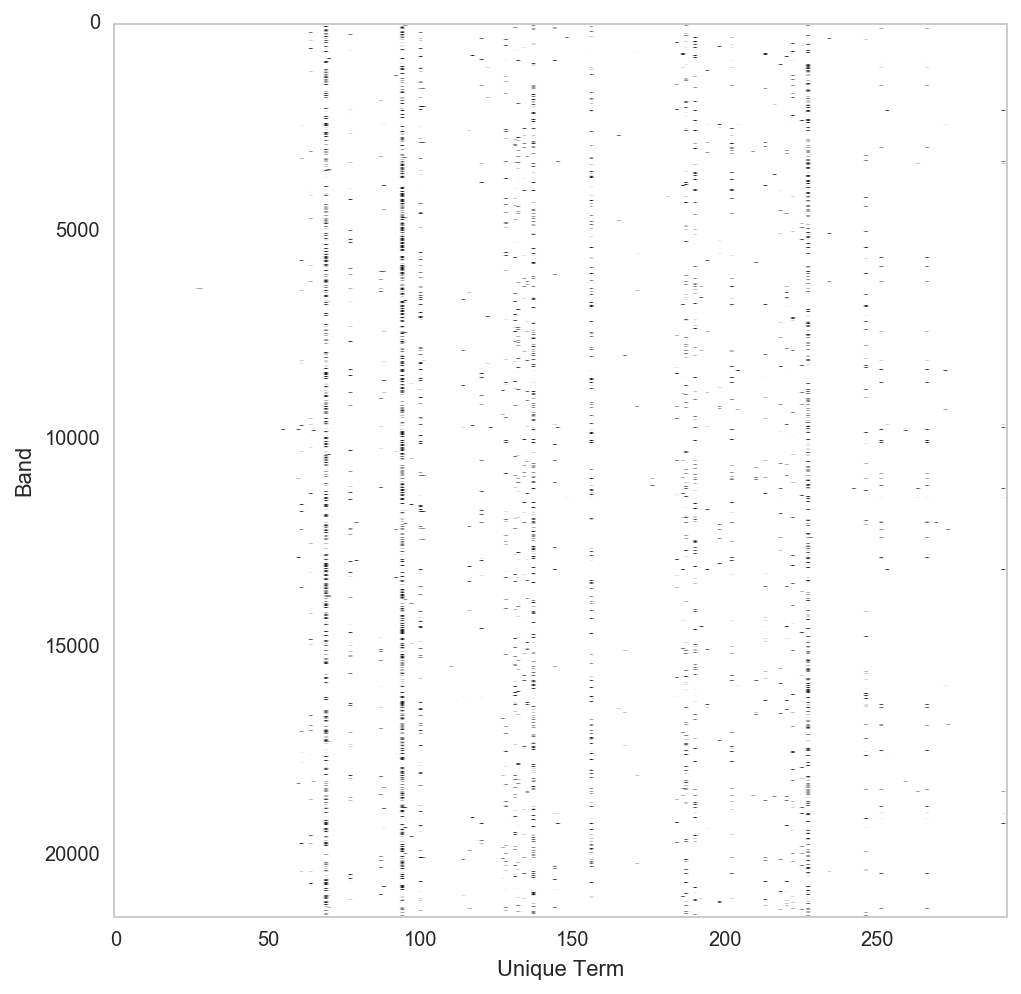The vertical trends we're seeing indicate genre terms repeated over bands. What this suggests should be obvious: some terms are more common than others. We can verify this by looking at the counts for the top genre terms that we extracted above and stored in genre_terms. The indices of the top terms should correspond with where the appear on the binary plot. Let's double check this for sanity.

In :
genre_terms.head(10)

Out:
Term TotalCount
94 Death 36633
69 Black 27528
227 Thrash 23336
137 Heavy 15421
156 Melodic 9495
100 Doom 7710
190 Progressive 7488
187 Power 6711
131 Grindcore 4031
202 Rock 3745
In :
popular_term_locations = genre_terms.index[:10]

fig, ax = plt.subplots(figsize=(8,8))
ax.imshow(binarized_terms, aspect='auto', origin='lower')
ax.grid('off')
ax.set_ylabel('Band')
ax.set_xlabel('Unique Term')
for location in popular_term_locations:
ax.axvline(location, lw=2, alpha=0.1)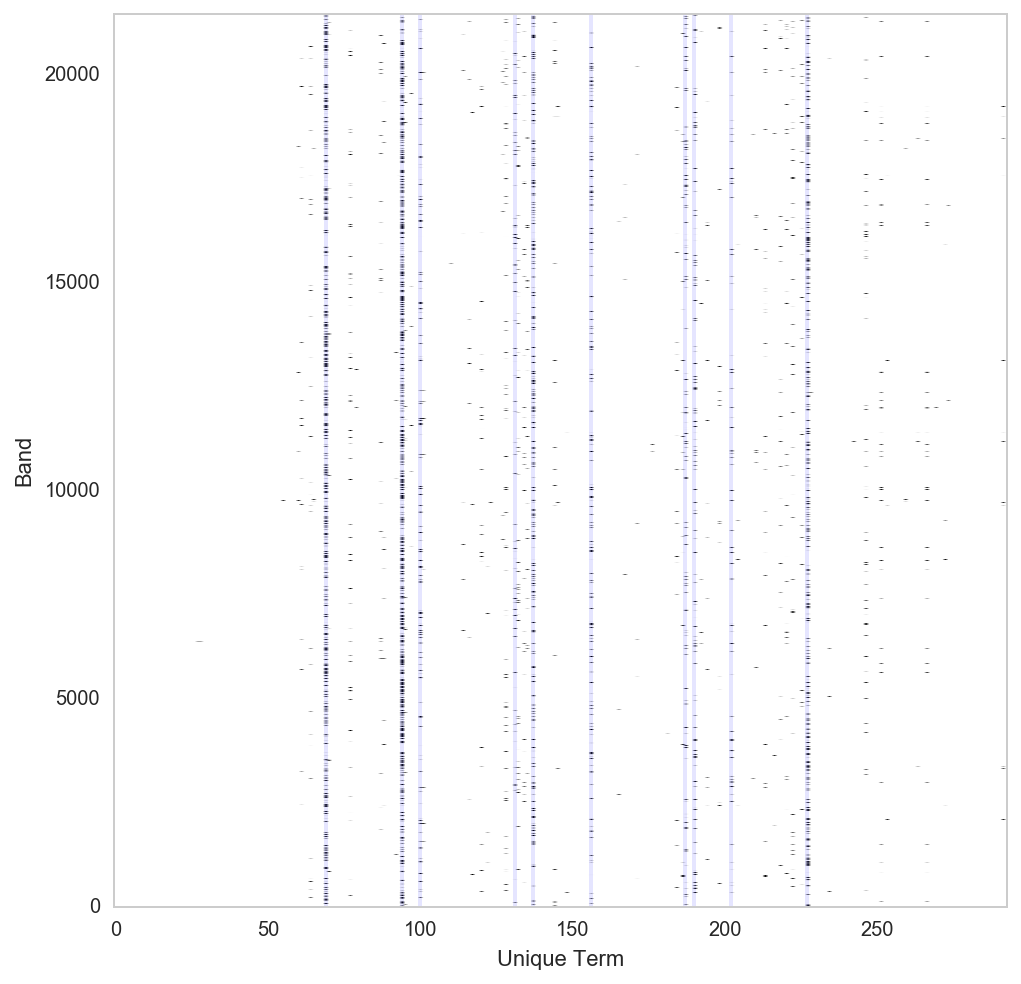The vertical lines we plotted do indeed line up with frequenly used genre terms. Sanity checked!

## Dimensionality reduction¶

As you can see our feature vector is one big matrix. The first step in almost any data exploration (IMO) should be to create a simple visualization of your data. Since each of our bands is described by a 293-dimension feature vector, some dimensionality reduction is in order.

### Principal Component Analysis (PCA)¶

While not technically an algorithm for dimensionality reduction (it's truly a decomposition algorithm), PCA can be used to find the axes in our feature space that capture the greatest amount of variance within the data. These axes are known as the principal components. Taking only the top components therefore is an effective way of "reducing" the dimensionality of your original data set.

In :
pca = PCA()
components = pca.fit_transform(binarized_terms)


Now we can visualize combinations of first three primary components to get an idea of any structure in our data set.

In :
fig, ax = plt.subplots(1,3,figsize=(14,4))
for i, (j, k) in enumerate([(0,1), (1,2), (0,2)]):
ax[i].scatter(components[:,j], components[:,k], alpha=0.05, lw=0)
ax[i].set_xlabel('Component %d' % (j+1))
ax[i].set_ylabel('Component %d' % (k+1))
fig.tight_layout()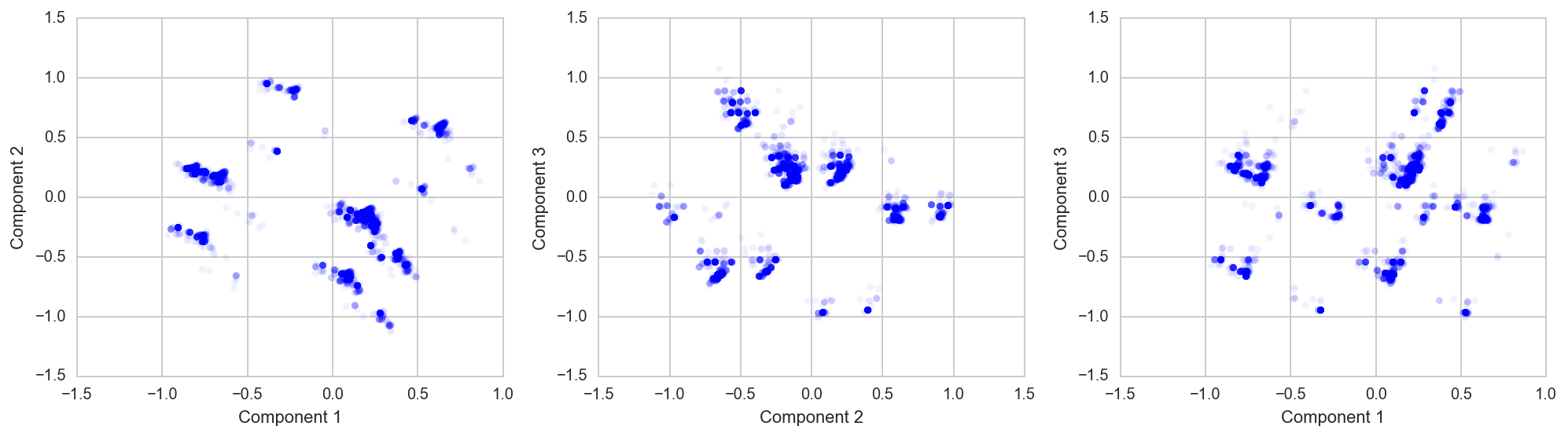There appears to be some structure revealed in the top principal components. Let's use matplotlib's hexbin to get an idea of the point density, which may not be apparent from the scatter plots.

In :
fig, ax = plt.subplots(figsize=(6,4))
hb = ax.hexbin(components[:,0], components[:,1], cmap=plt.cm.viridis, extent=(-1.5, 1, -1.5, 1.5), norm=LogNorm());
ax.set_xlabel('Component 1')
ax.set_ylabel('Component 2')
fig.colorbar(hb)

Out:
<matplotlib.colorbar.Colorbar at 0x7fd2ad1a9ef0>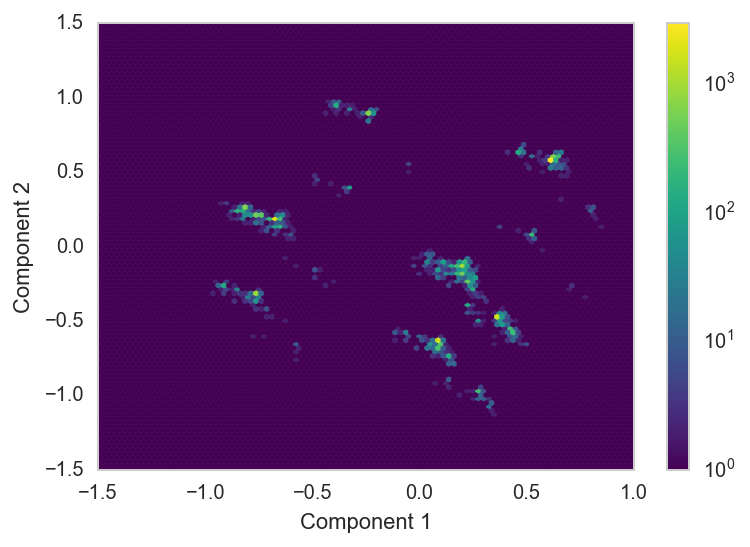In some regions there are nearly 10,000 overlapping data points! We definitely have distinct clusters on our hands. How can we effectively detect them?

## Clustering genres¶

Clustering will allow us to detect groups of similar data points. By clustering the results from our dimensionality reduction, we should be able to meaningfully partition our data into different groups.

### $k$-means¶

$k$-means is a relatively quick, low cost clustering algorithm that requires us to guess the number of clusters ($k$) beforehand (for an overview of how it works, you can check out my example implementation here). However we don't necessarily have a clear estimate for $k$. How many clusters are apparent in the hexbin plot above? 2? 6? More? The truth is that there is no correct answer.

One way to come up with an estimate is to run $k$-means with different values for $k$ and evaluate how well the clusters fit the shape of the data. We can then use the "hockey stick" method (that's a technical term) and choose the value for $k$ where the most significant bend occurs in the plot. The measure of how well the clusters fit is known as the inertia and reflects the variability within clusters. This isn't the only way to determine the optimal number of clusters, but it's what we'll use here. We'll run this using the first 3 principal components.

In :
distortions = []
for n in np.arange(1,11):
kmeans = KMeans(n_clusters=n)
clusters = kmeans.fit_predict(components[:,[0,1,2]])
distortions.append(kmeans.inertia_)

fig, ax = plt.subplots(figsize=(4,4))
ax.plot(np.arange(1,11), distortions, marker='o')
ax.set_xlabel('Number of clusters')
ax.set_ylabel('Inertia')
ax.set_ylim(0, ax.get_ylim());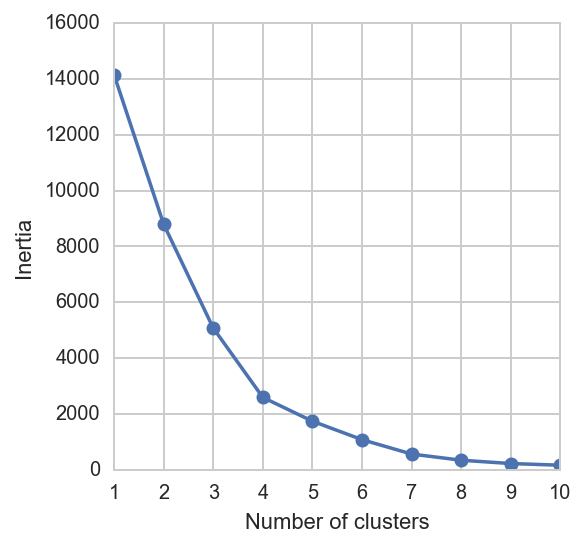Depending on what you think hockey sticks look like, $k$-means suggests either 4 or 7 clusters. Let's see what 4 clusters looks like.

In :
n_clusters = 4
kmeans = KMeans(n_clusters=n_clusters)
clusters = kmeans.fit_predict(components[:,[0,1,2]])

In :
fig, ax = plt.subplots(1,3,figsize=(14,4))
for i, (j, k) in enumerate([(0,1), (1,2), (0,2)]):
ax[i].scatter(components[:,j], components[:,k], alpha=0.1, lw=0, color=plt.cm.spectral(clusters/n_clusters))
ax[i].set_xlabel('Component %d' % (j+1))
ax[i].set_ylabel('Component %d' % (k+1))
fig.tight_layout()